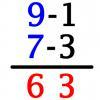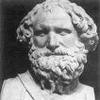#### You may also like### Vedic Sutra - All from 9 and Last from 10

Vedic Sutra is one of many ancient Indian sutras which involves a cross subtraction method. Can you give a good explanation of WHY it works?### Tournament Scheduling

Scheduling games is a little more challenging than one might desire. Here are some tournament formats that sport schedulers use.### Archimedes and Numerical Roots

The problem is how did Archimedes calculate the lengths of the sides of the polygons which needed him to be able to calculate square roots?

# Triangle Incircle Iteration

### Why do this problem?

This problem leads to a result which is easy to guess visually but not so easy to prove.
Geometrical, numerical, and algebraic ideas can all be used to reach a solution, and properties of averaging can also come out of the problem. Numerical patterns can be investigated using a spreadsheet.

### Possible approach

Use the interactivity (or accurately construct some triangles with their inscribed circles) and see what happens to the angles in the nested triangles.In order to see why this is happening, it's important to make sure everyone knows that the centre of the inscribed circle is at the point where the angle bisectors of the original triangle meet, and that radii meet tangents at a right angle. This information can be used to write expressions for the three angles in the new triangle in terms of the original angles.

Students could now create a spreadsheet which allows them to input three angles which sum to 180 degrees and use their expressions to work out the three new angles. By continuing the sequence, the angles quickly converge.

### Key questions

What seems to be happening to the angles in each new triangle that we draw?
How can we calculate the angles of each new triangle if we know the original angles?

### Possible extension

Investigate the sequence $(90-x/2), 90-(90-x/2)/2$ and so on to explain why the angles converge to their limit.
The three new angles are each the mean of a pair of the original angles. In general, what happens if you keep finding the mean of pairs of numbers to give three new numbers?

### Possible support

Work with numerical examples and try to explain the patterns formed.